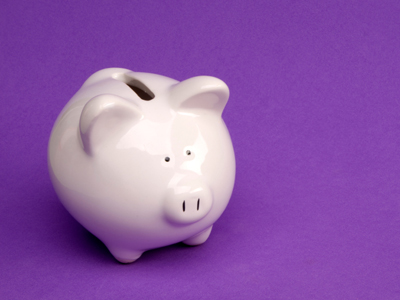If I put 20p into my piggy bank every day how long will it take me to save up £5?

# Year 2 Money - Addition and Subtraction

This quiz addresses the requirements of the National Curriculum KS1 Maths and Numeracy for children aged 6 and 7 in year 2. Specifically this quiz is aimed at the section dealing with solving simple problems in a practical context involving addition and subtraction of money of the same unit, including giving change.

Children in Year 2 should be familiar with adding and subtracting small amounts of money of the same unit. They should be able to use this skill to solve money problems which may involve finding a total or working out change.

This quiz will present your child with some practical problems on money of the same unit to help familiarise them with the concept.

Question 1
A chocolate bar costs 62p and a lolly costs 44p. How much do they cost altogether?
£1.60
£1.06
£1.07
£1.66
60p and 40p make £1 and 4p and 2p make 6p
Question 2
Kay has the following coins: £1, 10p, 50p and 5p.
How much does she have in total?
£1.75
£1.56
£1.65
£2.00
Counting the coins up from largest value to smallest is a good way to find a total
Question 3
Which of the following coins add up to 40p?
20p, 20p, 20p
10p, £1, 20p
5p, 20p, 20p, 5p
10p, 5p, 10p, 5p, 10p
Counting up in 10s could be useful
Question 4
Yoghurts cost 10p. If I buy 6, how much will it cost?
£6.00
16p
60p
66p
10 x 6 = 60
Question 5
I have saved £1.50 and my friend has saved 60p. How much have we saved altogether?
£2.50
£2.10
£1.21
£1.75
Picturing the 60p as a 50p and a 10p might help solve the problem
Question 6
Amy wants to buy an apple costing 20p. She has 12p. How much more does she need?
8p
32p
12p
18p
The difference between 12p and 20p is 8p
Question 7
I buy a hat for 92p and pay with two 50 pence coins. How much is my change?
8p
80p
£8
50p
Two 50p coins make £1. The difference between £1 and 92p is 8p
Question 8
I buy a toy for 43p and pay with a 50p coin. How much change will I receive?
7p
17p
27p
22p
The difference between 43 and 50 is 7
Question 9
A bus ticket costs 75p and a train ticket costs £1.10. How much more does the train ticket cost than the bus ticket?
£1.00
40p
35p
30p
Counting up from the smaller amount to the larger amount helps to find the difference
Question 10
Ben has £2 to spend. He buys a sweet costing 45p and a toy costing 50p. How much does he have left?
£1.50
50p
5p
£1.05
This is a two step problem - find the total first before working out the amount left